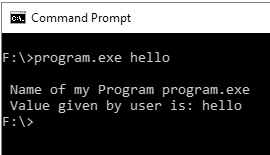## C Programming Tutorial Index

C allows passing values from the command line at execution time in the program. In this tutorial, you will learn about using command-line arguments in C.

The `main()` function is the most significant function of C and C++ languages. This `main()` is typically defined as having a return type of integer and having no parameters; something like this:

Example:

``````int main()
{
// body of the main() function
}``````

C allows programmers to put command-line arguments within the program, allowing users to add values at the very start of program execution.

## What are Command line arguments?

Command line arguments are the arguments specified after the program name in the operating system's command line. These argument values are passed to your program during execution from your operating system. To use this concept in your program, you have to understand the complete declaration of how the `main()` function works with the command-line argument to fetch values that earlier took no arguments with it (`main()` without any argument).

So you can program the `main()` in such a way that it can essentially accept two arguments where the first argument denotes the number of command line arguments and the second denotes the complete list of every command line argument. It is how you can code your command line argument within the parenthesis of `main()`:

Example:

``int main ( int argc, char  *argv [ ] )``

In the above statement, the command line arguments have been handled via the `main()` function, and you have set the arguments where

• argc (ARGument Count) denotes the number of arguments to be passed and
• argv [ ] (ARGument Vector) denotes a pointer array pointing to every argument that has been passed to your program.

You must make sure that in your command line argument, `argv` stores the name of your program, similarly, `argv` gets the pointer to the 1st command line argument that the user has supplied, and `*argv[n]` denotes the last argument of the list.

### Program for Command Line Argument

Example:

``````#include <stdio.h>

int main( int argc, char *argv [] )
{
printf(" \n Name of my Program %s \t", argv);

if( argc == 2 )
{
printf("\n Value given by user is: %s \t", argv);
}
else if( argc > 2 )
{
printf("\n Many values given by users.\n");
}
else
{
printf(" \n Single value expected.\n");
}
}``````

Output: